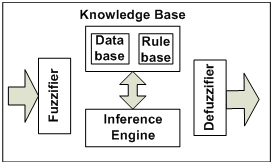Fuzzy rule-based systems (FRBS) are also known as fuzzy inference systems and fuzzy models. They are based on the fuzzy concept proposed by Zadeh in 1965 . It represents the reasoning of human experts in production rules (a set of fuzzy IF-THEN rules) to handle real-life problems from domains such as control [2, 3, 4], prediction and inference , data mining , bioinformatics data processing , etc.

FRBSs are a competitive alternative to other classic models and algorithms in order to solve classification and regression problems. Generally, an FRBS consists of four functional parts (see Figure 1):

• a fuzzification interface (fuzzifier) which transforms the crisp inputs into degrees of membership function of the fuzzy term of linguistic variable.
• a knowledge base composed of a database and a rulebase. While the database includes the fuzzy set definitions,
• the rulebase contains the fuzzy IF-THEN rules. We will represent the knowledge as a set of rules. Each one has the following structure.

IF premise (antecedent), THEN conclusion (consequent).

• an inference engine which performs the inference operations on the fuzzy IF-THEN rules. There are two kinds of inference for fuzzy systems based on linguistic rules: The Mamdani and the Takagi Sugeno Kang model.
• a defuzzification (defuzzifier) process to obtain the crisp values. There are several methods for defuzzification such as the weighted average, centroid, etc.Figure 1. The components of FRBS

References

 L.A. Zadeh, "Fuzzy sets", Information and Control, vol. 8, pp. 338 – 353, 1965.

 C.C. Lee, "Fuzzy Logic in control systems: Fuzzy Logic controller part I", IEEE Trans. Syst., Man, Cybern., vol. 20, no.2, pp. 404-418, 1990.

 C.C. Lee, "Fuzzy Logic in control systems: Fuzzy Logic controller part II", IEEE Trans. Syst., Man, Cybern., vol. 20, no.2, pp. 419-435, 1990.

 W. Pedrycz, "Fuzzy Control and Fuzzy Systems," New York: Wiley, 1989.

 Pei-Chann Chang, Chen-Hao Liu, “A TSK type fuzzy rule based system for stock price prediction, Expert Systems with Applications,” Volume 34, Issue 1, pp 135-144, ISSN 0957-4174, 2008.

 Li-Xin Wang, “The WM method completed: a flexible fuzzy system approach to data mining,” IEEE Transaction on Fuzzy Systems, Vol 11 (6), pp. 768 – 782, 2003.

 Bill C.H. Chang and Saman K. Halgamuge, “Protein motif extraction with neuro-fuzzy optimization,” Bioinformatics 18 (8): pp. 1084-1090, 2002.